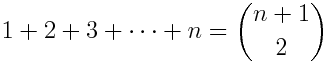# Triangular numbers and simplices

A couple weeks ago I posted a visual proof thatThis says that the nth triangular number equals C(n+1, 2), the number of ways to choose two things from a set of n + 1 things.

I recently ran across a similar proof here. A simplex is a generalization of a triangle, and you can prove the equation above by counting the number of edges in simplices.

A 0-simplex is just a point. To make a 1-simplex, add another point and connect the two points with an edge. A 1-simplex is a line segment.

To make a 2-simplex, add a point not on the line segment and add two new edges, one to each vertex of the line segment. A 2-simplex is a triangle.

To make a 3-simplex, add point above the triangle and add three new edges, one to each vertex of the triangle. A 3-simplex is a tetrahedron.

Now proceed by analogy in higher dimensions. To make an n-simplex, start with an n-1 simplex and add one new vertex and n new edges. This construction shows that the number of edges in an n simplex is 1 + 2 + 3 + … + n.

Another way to count edges is to note that an n-simplex has n+1 vertices and an edge between every pair of vertices. So an n simplex has C(n+1, 2) edges. So C(n+1, 2) must equal 1 + 2 + 3 + … + n.

## 6 thoughts on “Triangular numbers and simplices”

1. For more than you could ever possibly want to know about triangular numbers and their generaliations, have a look at “Figurate Numbers” by Deza Michel et al.

2. Interesting that C(n+1,2) = sum(1..n)

I was wondering if there is there a general algorithm / formula to determine C(n,m)? My math is 1/10,000th of yours, so please keep it simple :)

3. Katz: The simplest case is C(n, m) = n! /(m! (n-m)!). See this page for fine print and generalizations.

4. Cool, thank you very much for that help John.

5. Dave Tate

There’s an almost-visual proof of this attributed to the child Gauss, who when asked (as busy work to shut him up) to add the numbers from 1 to 100, thought for a moment and said “5050”. According to this story, he had noted that the numbers {1, 2, …, 100} can be grouped as pairs (1,100), (2, 99), … (50, 51) — fifty pairs, each adding to 51, so 50*51 = 5050.

For the general case, if n is even, you can write 1+2+…+n as (1+n)+(2+n-1)+…+(n/2+1+n/2) = n*(n+1)/2 = C(n+1,2).
If n is odd, note that 1+…+(n-1) = C(n,2) by the above, and C(n,2) + n = (n^2 – n + 2n)/2 = (n^2 + n)/2 = n(n+1)/2 = C(n+1,2).

The key step, though, is the initial grouping into pairs, which (for me at least) is more visual than symbolic/algebraic.

6. Dave Tate

Did I really say 50*51 = 5050? Sheesh. 50*101, of course. Now I must go do penance.